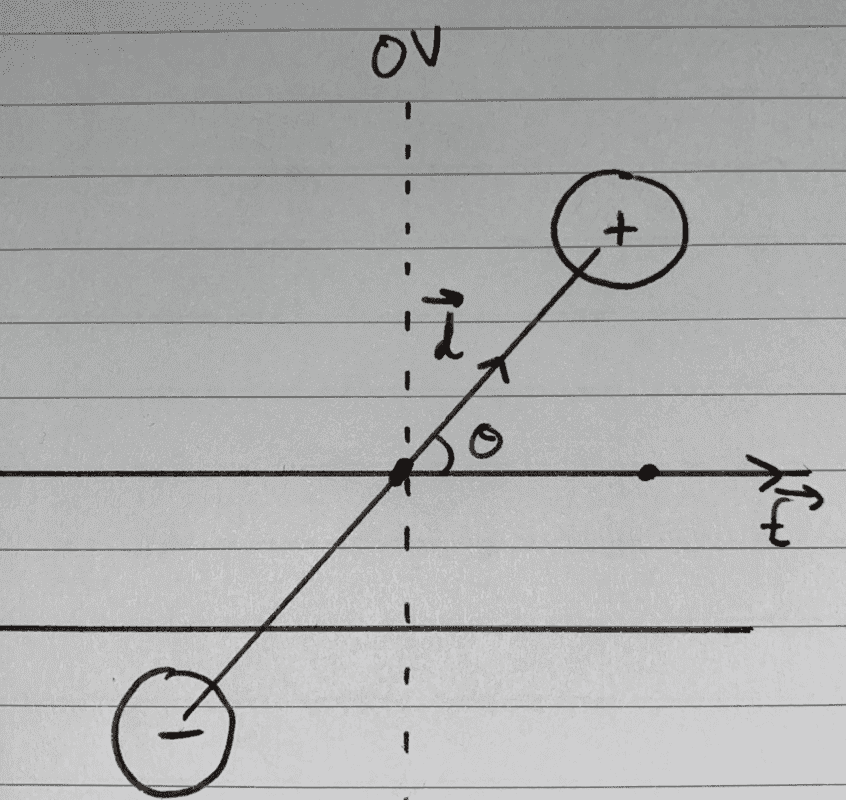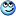# Potential energy of a dipole in an external field

I'm considering the arrangement shown below. Let the positive charge be ##q##, and the negative be ##-q##. To derive the potential energy of this configuration, one usually adds the potential energies of both of the charges in the external field, taking the zero volts equipotential of the external field to be along the line at ##\theta = \frac{\pi}{2}##.

##U = qV_{1} - qV_{2} = q \times -\frac{Ed}{2}\cos{\theta} - q \times \frac{Ed}{2}\cos{\theta} = -E(qd)\cos{\theta} = -\vec{E} \cdot \vec{p}## where ##\vec{p} = q\vec{d}##.My question is, why isn't the potential energy of the two charges ##U_{3} = -\frac{q^{2}}{4\pi\epsilon_{0}d^{2}}## also included in the summation above?

Since, for any collection of charged particles, the potential energies of subsystems can be added pairwise (i.e. ##-\Delta U = (W_{12} + W_{21}) + (W_{13} + W_{31}) + (W_{23} + W_{32}) = -\Delta U_{a} - \Delta U_{b} - \Delta U_{c}##. And to me, there seem to be three charged things at play here: the source (whatever it happens to be, e.g. perhaps an infinite charged sheet), and the two charges.

Consequently, the potential energy should be the sum of that of the source and +ve charge, the source and -ve charge, and the two charges. Although I think this might be incorrect since I haven't defined ##0V## to be at infinite distance!

Gold Member
2022 Award
##U_3## is simply a constant and doesn't do anything to the dynamics of the dipole in the external field. As any additive constants in the energy, they are physically insignifant.

That's a great gift in this context, because otherwise you'd also need the energy for each point charge itself, and this diverges. You can simply subtract this diverging infinite "self-energy".

That's the first place in physics where you renormalize. Renormalization thus not only occurs in quantum field theory but already in classical field theory. The only trouble with the classical theory is that for accelerating point charges there's no satisfactory exact solution for the problem of infinities. The best we can do is to use the Landau-Lifshitz prescription to cure the oddities of the (in)famous Abraham-Lorentz-Dirac equation for the motion of charged particles including radiation reaction.

•etotheipi
Homework Helper
Gold Member
My question is, why isn't the potential energy of the two charges ##U_{3} = -\frac{q^{2}}{4\pi\epsilon_{0}d^{2}}## also included in the summation above?

Correction: ##U_3=-\dfrac{q^{2}}{4\pi\epsilon_{0}d}.##

•etotheipi
Correction: ##U_3=-\dfrac{q^{2}}{4\pi\epsilon_{0}d}.##

Yep, bad mistakeGold Member
To derive the potential energy of this configuration, one usually adds the potential energies of both of the charges in the external field, taking the zero volts equipotential of the external field to be along the line at θ=π2θ=π2\theta = \frac{\pi}{2}.

You are calculating the work it takes to rotate the dipole in the given field.

My question is, why isn't the potential energy of the two charges [...] also included in the summation above?

This is the work done to assemble the dipole in the absence of the given field.

You could add the two together if you were interested in calculating the work it takes to assemble the dipole in the absence of any external field, and then to rotate it in the given field.

••vanhees71 and etotheipi